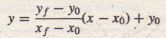Interpolation

Engineering problems often require the analysis of data pairs. For example, the paired data might represent a cause and effect, or input-output relationship, such as the current produced in a resistor as a result of an applied voltage, or a time history, such as the temperature of an object as a function of time. Another type of paired data represents a profile, such as a road profile (which shows the height of the road along its length). In some applications we want to estimate a variable’s value between the data points. This process is called interpolation. In other cases we might need to estimate the variable’s value outside of the given data range. This process is extrapolation. Interpolation and extrapolation are greatly aided by plotting the data. Such plots, some perhaps using logarithmic axes, often help to discover a functional description of the data.
Suppose that x represents the independent variable in the data (such as the applied voltage in the preceding example), and y represents the dependent variable (such as the resistor current). In some applications the data set will contain only . one value of y for each value of x. In other cases there will be several measured values of y for a particular value of x. This condition is called data scatter. For example, suppose we apply 10 V to a resistor, and measure 3.1 mA of current. Then, repeating the experiment, suppose We measure 3.3 mA the second time. If we average the two results, the resulting data point will be x = 10V,y = 3.2 mA, which is an example of aggregating the data, In this section we assume that the . data have been aggregated if necessary, so only one value of y corresponds to a specific value of x. You can use the methods of Sections 7.1 and 7.2 to aggregate the data by computing its mean. The data’s standard deviation indicates how much the data is spread around the aggregated point.

Suppose we have the following temperature measurements, taken once an hour starting at 7:00 A.M. The measurements at 8 A.M. and 10 A.M. are missing for some reason, perhaps because of equipment malfunction.

Time                                7 A.M. 9 A.M. 11 A.M. 12 noon
Temperature (ºF)             49       57         71            75

A plot of this data is shown in Figure 7.4-1 with the data points connected by, dashed lines. If we need to estimate the temperature at 10 A.M., we can read the value from the dashed line that connects the data points at 9 A.M. and 11A.M. From the plot wethus estimate the temperature at 8 A.M. to be 53°F and at 10 A.M. to be 64°F. We have just performed linear interpolation on the data to obtain an estimate of the missing data. Linear interpolation is so named because it is equivalent to connecting the data points with a linear function (a straight line).

Of course we have no reason to believe that the temperature follows the straight lines shown in the plot, and our estimate of 64°F will most likely be incorrect, but it might be close enough to be useful. When using interpolation, we must always keep in mind that our results will be approximate and should be used with caution. In general, the more closely spaced the data, the more accurate the interpolation. Plotting the data sometimes helps to judge the accuracy of the interpolation.

Using straight lines to connect the data points is the simplest form of interpolation. Another function could be used if we have a good reason to do so. Later in this section we use polynomial functions to do the interpolation,

Linear interpolation in MATLAB is obtained with the interp1 and interp2 functions. Suppose that x is a vector containing the independent variable data and that y is a vector containing the dependent variable data. If x_int is a vector containing the value or values of the independent variable at which we wish to estimate the dependent variable, then typing interp1 (x,y , x_int)

produces a vector the same size as x_int containing the interpolated values of y that correspond to x_into For example, the following session produces an estimate of the temperatures at 8 A.M. and 10 A.M. from the preceding data. The vectors x and y contain the times and temperatures, respectively.

» x = [7, 9, 11, 12];
» y = [49, 57, 71, 75);
» x_int = [8, 10];
» interp1 (x,y,x_int)
ans =
53
64

You must keep in mind two restrictions when using the interp1 function .. The values of the independent variable in the vector x must be in ascending order; and the values in the interpolation vector x_int must lie within the range of the values in X. Thus we cannot use the interp1 function to estimate the temperature. at 6 A.M., for example.

The interp1 function can be used to interpolate in a table of values by defining y to be a matrix instead of a vector. For example, suppose we now have temperature measurements at three locations and that the measurements at 8 A.M. and 10 A.M. are missing for all three locations. The data is

Temperatures (ºF)

Time     Location 1     Location 2     Location 3
7 A.M.         49                    52                 54
9 A.M.         57                    60                 61
11 A.M.       71                    73                 75
12 noon      75                    79                  81

We define x as before, but now we define y to be a matrix whose three columns contain the second, third, and fourth columns of the preceding table. The following session produces an estimate of the temperatures at 8 A.M. and 10 A.M. at each location.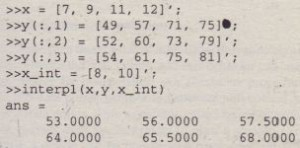Th~s the estimated temperatures at 8 A.M. at each location are 53,56, and 57.5°F, respectively. At 10 A.M. the estimated temperatures are 64, 65.5, and 68. From this example we see that if the first argument x.in the interp1 (x , y, x_int) function is a vector and the second argument y is a matrix, the function interpolates . between the rows of y and computes a matrix having the same number of columns as y and the same number of rows as the number of values in x_int.

Note that we need not define two separate vectors x and y. Rather, we can define a single matrix that contains the entire table. For example, by defining the matrix temp to be the preceding table, the session would look like this:Two-Dimensional Interpolation

Now suppose that we have temperature measurements at four locations at 7 A.M. These locations are at the comers of a rectangle 1 mi wide and 2 mi long. Assigning a coordinate system origin (0, 0) to the first location, the coordinates of the other locations are (1, 0), (l, 2), and (0, 2); see Figure 7.4-2. The temperature measurements are shown in the figure. The temperature is a function of two variables, the coordinates x and y. MATLAB provides the interp2 function to interpolate functions of two variables. If the function is written as z = f(x, y) and we wish to estimate the value of z for x = X¹ and y¹ = y², the syntax is interp2 (x,y,z,x_i,y_i).

Suppose we want to estimate the temperature at the point whose coordinates are (0.6, 1.5). Put the x coordinates in the vector x and the y coordinates in the vector y. Then put the temperature measurements in a matrix z such that going across a row represents an increase in x and going down a column represents an increase in y. The session to do this is as follows:

» x  [0,1];
» y  [0,2];
» z  [49,54;53,57]
z  =
49   54
53   57
» interp2 (x,y,z,0.6,1,5)
ans =
54.5500

Thus the estimated temperature is 54.55°.

The syntax of the interp1 and interp2 functions is summarized in Table 7.4-1.MATLAB also provides the interpn function for interpolating multidimensional arrays.

Cubic-Spline Interpolation

We have seen that the use of high-order polynomials can exhibit undesired behavior between the data points, and this behavior can make high-order polynomials unsuitable for interpolation. An alternative procedure that is widely used is to fit the data points using a lower-order polynomial between each pair of adjacent data points. This method is .called spline interpolation and is so named for the splines used by illustrators to draw a smooth curve through a set of points. Such a device can be constructed from a length of lead coated with rubber or flexible plastic. It can be bent to provide a drawing guide passing through the data points.

Spline interpolation obtains,an exact fit that is also smooth. The most common procedure uses cubic polynomials, called cubic splines, and thus is called cubic-spline interpolation. If the data is given as n pairs of (x, y) values, then n – I cubic polynomials are used. Each has the formfor xi ≤ x ≤  and i = 1,2, … , n – 1. The coefficients ai, bi, ci, and di, for each polynomial are determined so that the following three conditions are satisfied for each polynomial:

1. The polynomial must pass through the data points at its endpoints at x; and
xi + 1·
2. The slopes of adjacent polynomials must be equal at their common data point
3. The curvatures of adjacent polynomials must be equal at their common data point

For example, a set of cubic splines for the temperature data given earlier follows (y represents the temperature values, and x represents the hourly values). The data is repeated here.

x :   7   9    11 12
y :  49 57  71 75

We will shortly see how to use MATLAB to obtain these polynomials. For 7 ≤ x ≤ 9,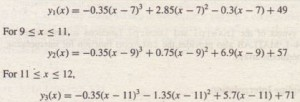MATLAB provides the spline command to obtain a cubic-spline interpolation. Its syntax is y_in t = sp 1ine (x , y , x_in t ) , where x and y are vectors containing the data and x_int is a vector containing the values of the independent variable x at which we wish to estimate the dependent variable Y: The resulty _int is a vector the same size as x_int containing the interpolated values of Y that correspond to x_into The spline fit can be plotted by plotting the vectors x_i n t and y_i n t. For example, the following session produces and plots a cubic-spline fit to the preceding data, using an increment of 0.01 in the x values.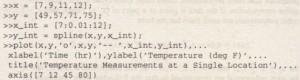The plot is shown in Figure 7.4-3. The dashed lines represent linear interpolation, and the solid curve is the cubic spline. Note that if we use the spline plot to estimate the temperature at 8 A.M., we obtain approximately 51°F. If we evaluate the spline polynomial at x = 8, we obtain y(8) = 51.2°F. In either case the estimate is different from the 53°F estimate obtained from linear interpolation. It is impossible to say which estimate is more accurate without having more understanding of the temperature dynamics.

Instead of plotting the cubic spline and estimating a point on the plot, we can obtain a more accurate calculation, more quickly, by using the following variation of the interp1 function.

y_est = interp1 (x,y,x_est, ‘spline’)

In this form the function returns a column vector y.est that contains the estimated values of y that correspond to the x values specified in the vector x_e s t, using cubic-spline interpolation. For example, to estimate the value of y at x = 8 for the preceding data. we would type interp1 (x, y, 8, ‘ spline’ ). MATLAB ‘returns the answer 51.2. To estimate the value of y at two different points, say, x = 8 and x = 10, we would type interp1 (x,y, [8, 10], ,spline; ) MATLAB returns the answers 51.2 and 64.3.
In some applications it is helpful to know the polynomial coefficients, but we cannot obtain the spline coefficients from the interp1 function. However, we can use the form.

(breaks, coeffs, m, n) = unmkpp(spline(x,y))

to obtain the coefficients of the cubic polynomials.The vector breaks contains the x values of the data. and the matrix ‘coef f s is an m x n matrix containing the coefficients of the polynomials. The scalars m and n give the dimensions of the matrix coeffs the number of polynomials, and n is the number of coefficients for each polynomial (MATLAB will fit a lower-order polynomial if possible, so ‘there can be fewer than four coefficients). For example, using the same data, the following session produces the coefficients of the polynomials given earlier: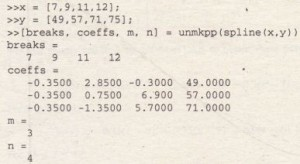The first row of the matrix coeffs contains the coefficients of the first polynomial, and so on. These functions are summarized in Table 7.4-2. The Basic Fitting interface, which is available on the Tools menu of the Figure window, can be used for cubic-spline interpolation. See Chapter 5, Section 5.7 for instructions for using the interface.

Application to Robot Control

Splines are used to control robotic devices such as the arm shown in Figure 7.4-4. This particular arm is a simple one that moves in a two-dimensional plane. It has a motor at its “shoulder” and a motor at its “elbow.” When these two motors rotate through the proper angles, the arm can place its hand at a desired point in the plane. Robots have a supervisory computer that accepts user input, such as the desired location of the hand. The computer then calculates how much rotation  each motor must produce to move the hand from its starting point to its desired final position. Then, at regular time intervals, the supervisory computer feeds the angular motion commands to the two smaller computers that control each motor. In many devices the supervisory computer uses cubic-spline inter- polation to generate the angular motion commands. The advantage of a cubic spline is that it results in smooth robot motion. We now show how the splines are computed.Figure 7.4-4 A robot arm having two joints. (a) Dimensions and arm angles. (b) The hand’s path is not a straight line, but a complicated curve.

We can use trigonometry to obtain the following expressions for the coordinates of the elbow and the hand. For the elbow, X elbow = L\ cos 0\ and Y elbow = L¹ sinOI. The hand’s coordinates (x, y) are given by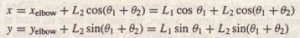These two equations can be solved for θ1 and θ2 in terms of x and Y so that we can determine the elbow and arm angles necessary to place the hand at a specified position given by x and y. We omit the details of the solution and just state the results.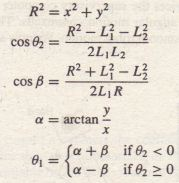If we use a cubic polynomial to express the joint angles as functions of time for 0 ≤ t ≤ T, then we can specify four conditions for each angle. Two of these conditions should require the polynomial to pass through the starting value θ(0) and the-ending value θ(T). The other two conditions require that the slope of the polynomial should be 0 at the start and finish because we want the robot to start from rest and to finish at rest. The cubic polynomial that satisfies these conditions is

θ (t) = at³ +bt² +θ (0)

where a = 2[θ (0) – θ (T)] / T³ and b = -3 [ θ (0) – θ (T)] / T²

For example, suppose-the .robot arm shown in Figure 7.4-4a has the lengths L \ = 4 and L2 = 3 ft. Suppose also that in 2 sec we want the hand to move from x(0) = 6.5, y(0) = 0 to x(2) = 0, y(2) == 6.5 ft. We obtain the following angle solutions from equations (1.4-3) through (7.4-7): 01(0) = -18.717°,0\(2) = 71.283°, and 02(0)= 02(2) = 44.049° e The polynomials. If the robot controller uses these polynomials to generate commands to the motors, ·then the path of the hand looks like that shown in Figure 7.4-4b. Note that the hand’s path is not a straight line between the starting and stopping points, and this motion might result in a collision with nearby objects.

In many applications we want to control the hand’s path more precisely. For example; the robot might be used for welding along a specific path. Suppose we want the path to be a straight line. Then we must specify a series of intermediate .locations for the hand to pass through. These locations must lie on the straight the connecting the starting and ending hand positions. These points are called points. To obtain smooth motion, we want the hand to pass through these point without stopping or changing speed, so we use cubic splines to interpolate between these points ..If the hand is initially at the point (x0, y0) and we want it to move to (xI, YI) in a straight line, the line`s equation -is